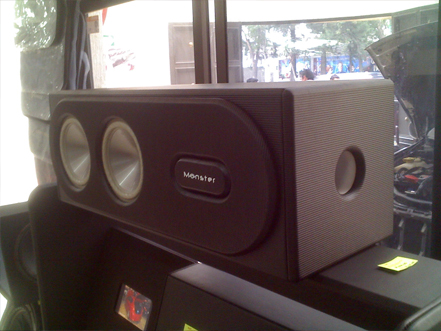8" subwoofers Fb=35Hz Vb=2 cu feet Port= 3" x L=14" each one8" subwoofers Fb=35Hz Vb=2 cu feet Port= 3" x L=14" each one8" subwoofers Fb=35Hz Vb=2 cu feet Port= 3" x L=14" each one8" subwoofers Fb=35Hz Vb=2 cu feet Port= 3" x L=14" each one8" subwoofers Fb=35Hz Vb=2 cu feet Port= 3" x L=14" each one8" subwoofers Fb=35Hz Vb=2 cu feet Port= 3" x L=14" each one8" subwoofers Fb=35Hz Vb=2 cu feet Port= 3" x L=14" each one8" subwoofers Fb=35Hz Vb=2 cu feet Port= 3" x L=14" each one8" subwoofers Fb=35Hz Vb=2 cu feet Port= 3" x L=14" each one8" subwoofers Fb=35Hz Vb=2 cu feet Port= 3" x L=14" each one8" subwoofers Fb=35Hz Vb=2 cu feet Port= 3" x L=14" each onePW8
Ricardo Rangel: rangel_ldi@hotmail.com
Object Designer México, Mexico